Get inspired by the success stories of our students in IIT JAM MS, ISI  MStat, CMI MSc DS.  Learn More

# Kernel of a linear transformation | ISI MStat 2016 Problem 4 | PSB SampleThis is a beautiful problem from ISI MStat 2016 Problem 4 PSB (sample) based on Vector space. It uses several concepts to solve it. We provide a detailed solution with prerequisites mentioned explicitly.

## Problem- ISI MStat 2016 Problem 4

For each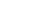define a function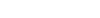by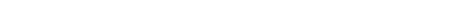For everyfind the dimension of the null space of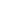.

## Prerequisites

• kernel or Null space of a linear transformation
• Dimension
• Spanning & Linearly Independent vectors of a vector space

## Solution

Here we have to find the Kernel or null space ofi.e {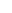: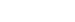} .is defined asSo,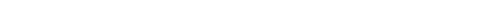, which gives

(i)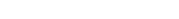if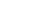(ii)if(iii)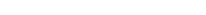(iv)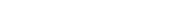(iii) & (iv)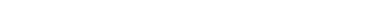ifAnd if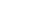thenif c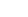.

Now for different values of c and using (i),(ii),(iii) and (iv) we will find the Null space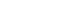as follows ,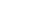=Therefore for different values of c we will get different dimension ofas follows ,

If c=-1 then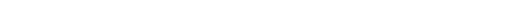. Hence the vectors {(1,0,0,0) , (0,1,1,0) ,(0,0,0,1) } spansand they are Linearly Independent . Thus on this case dimension of null spaceis 3 .

If c=0 then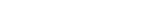. Thus on this case dimension of null spaceis 0.

If c=1 then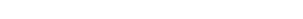.Hence the vectors { (0,1,-1,0) } spansand they are Linearly Independent . Thus on this case dimension of null spaceis 1 .

Finally if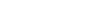then. Thus on this case dimension of null spaceis 0.

This is a beautiful problem from ISI MStat 2016 Problem 4 PSB (sample) based on Vector space. It uses several concepts to solve it. We provide a detailed solution with prerequisites mentioned explicitly.

## Problem- ISI MStat 2016 Problem 4

For eachdefine a functionbyFor everyfind the dimension of the null space of.

## Prerequisites

• kernel or Null space of a linear transformation
• Dimension
• Spanning & Linearly Independent vectors of a vector space

## Solution

Here we have to find the Kernel or null space ofi.e {:} .is defined asSo,, which gives

(i)if(ii)if(iii)(iv)(iii) & (iv)ifAnd ifthenif c.

Now for different values of c and using (i),(ii),(iii) and (iv) we will find the Null spaceas follows ,=Therefore for different values of c we will get different dimension ofas follows ,

If c=-1 then. Hence the vectors {(1,0,0,0) , (0,1,1,0) ,(0,0,0,1) } spansand they are Linearly Independent . Thus on this case dimension of null spaceis 3 .

If c=0 then. Thus on this case dimension of null spaceis 0.

If c=1 then.Hence the vectors { (0,1,-1,0) } spansand they are Linearly Independent . Thus on this case dimension of null spaceis 1 .

Finally ifthen. Thus on this case dimension of null spaceis 0.

This site uses Akismet to reduce spam. Learn how your comment data is processed.

### Knowledge Partner